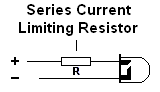# LED Current Limiting Resistor

LEDs (Light Emitting Diodes) must always be operated with a resistor in series to limit the current to a safe level. Most LEDs can only tolerate a current of about 30mA maximum. To calculate the resistor value required the forward voltage (Vf) and current (If)of the LED must be known. These can be found from the suppliers catalogue. For standard LEDs Vf is about 2V and If is about 20mA.The resistor value can be calculated as follows:

R = (Vs - Vf)  / If

= (Supply Voltage - Forward Voltage) / Forward Current

Note that If is in Amps, NOT Milliamps! (20mA = 0.02A)